#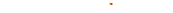###### Rotate Texture 1 and Texture 2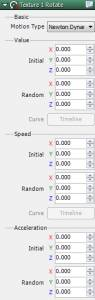Basics Motion Type
 Constant Set fixed angle Newton Dynamics Rotate at speed and acceleration with respect to each axis. Curve Value Set fixed angle for keyframes in Timeline. Curve Speed Set rotational speed for keyframes in Timeline.

Constant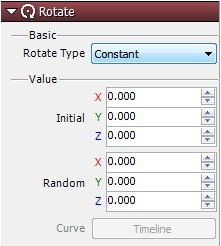Set to Constant to keep the initial angle from moving. Compared to other types of functions, one of the characteristics of Constant is the processing load on the CPU is light.

Newton Dynamics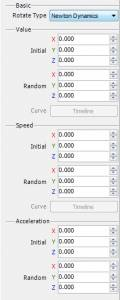Set the rotational speed and acceleration for each axis. Set each axis to a random range.
 Value Specify initial position of texture. The random value is the initial position with a random range. The random range is between – (specified value) and + (specified value). Speed Specify the rotational speed of texture. The random value is the loss range of rotational speed. The random range is between – (specified value) and + (specified value). Acceliration Set rotational acceleration of texture. The random value is the loss range of rotational acceleration. The random range is between – (specified value) and + (specified value).

Curve Value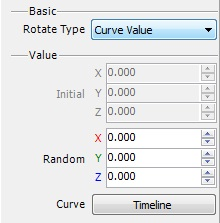Set fixed rotation position for graphs in Timeline. Change keyframes in realtime as keyframes run.

Curve Speed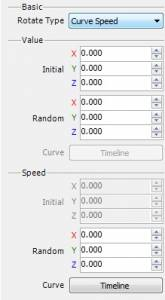Set rotational speed for graphs in Timeline. Change keyframes in real time as keyframes run. Also set initial angles.